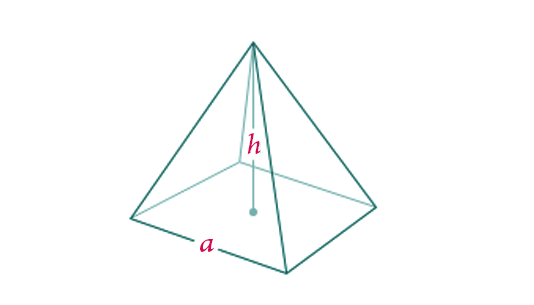# Volume of a Square Pyramid Formula

A square pyramid is a type of pyramid with a square-shaped base and 4 triangular faces which meet each other at a vertex. The volume of this pyramid can be found using the formula given below.The Volume of a Square Pyramid Formula is =

$$\begin{array}{l}\frac{1}{3}\end{array}$$
$$\begin{array}{l}a^{2}\end{array}$$
h

Where,
is the base length of the square base of the square pyramid.
h is the height of the square pyramid.

### Solved Example

Question: Find the volume of a square pyramid of base length 6 cm and height 8 cm.
Solution:
Given,

a = 6 cm
h = 8 cm
Volume of a square pyramid
=
$$\begin{array}{l}\frac{1}{3}\end{array}$$
a
2h
=
$$\begin{array}{l}\frac{1}{3}\end{array}$$
$$\begin{array}{l}\times\end{array}$$
(6 cm)2
$$\begin{array}{l}\times\end{array}$$
8 cm
=
$$\begin{array}{l}\frac{1}{3}\end{array}$$
$$\begin{array}{l}\times\end{array}$$
36 cm2
$$\begin{array}{l}\times\end{array}$$
8 cm
= 96 cm3